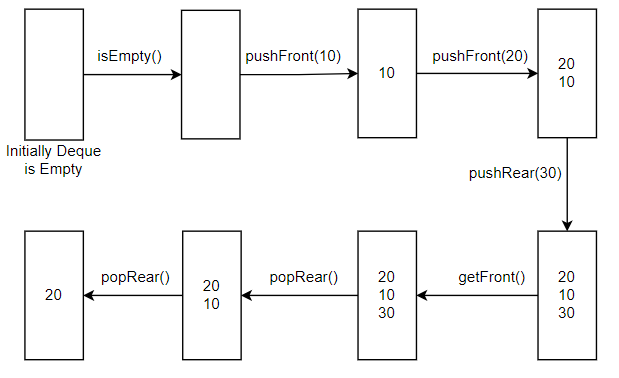New update is available. Click here to update.

# Implement Deque

Contributed by
Shivam
Last Updated: 23 Feb, 2023
Easy0/40
Avg time to solve 15 mins
Success Rate 85 %Share28 upvotes

## Problem Statement

Suggest Edit

#### Design a data structure to implement deque of size ‘N’. It should support the following operations:

``````pushFront(X): Inserts an element X in the front of the deque. Returns true if the element is inserted, otherwise false.

pushRear(X): Inserts an element X in the back of the deque. Returns true if the element is inserted, otherwise false.

popFront(): Pops an element from the front of the deque. Returns -1 if the deque is empty, otherwise returns the popped element.

popRear(): Pops an element from the back of the deque. Returns -1 if the deque is empty, otherwise returns the popped element.

getFront(): Returns the first element of the deque. If the deque is empty, it returns -1.

getRear(): Returns the last element of the deque. If the deque is empty, it returns -1.

isEmpty(): Returns true if the deque is empty, otherwise false.

isFull(): Returns true if the deque is full, otherwise false.
``````

#### Following types of queries denote these operations:

``````Type 1: for pushFront(X) operation.
Type 2: for pushRear(X) operation.
Type 3: for popFront() operation.
Type 4: for popRear() operation.
Type 5: for getFront() operation.
Type 6: for getRear() operation.
Type 7: for isEmpty() operation.
Type 8: for isFull() operation.
``````
Detailed explanation ( Input/output format, Notes, Images )##### Constraints:
``````1 <= N <= 1000
1 <= Q <= 10^5
1 <= P <= 8
1 <= X <= 10^5

Time Limit: 1 sec

Where ‘N’ represents the size of the deque, ‘Q’ represents the number of queries, ‘P’ represents the type of operation and ‘X’ represents the element.
``````
##### Sample Input 1:
``````5 7
7
1 10
1 20
2 30
5
4
4
``````
##### Sample Output 1:
``````True
True
True
True
20
30
10
``````
##### Explanation 1:
``````For the given input, we have the number of queries, Q = 7.
Operations performed on the deque are as follows:

isEmpty(): Deque is initially empty. So, this returns true.
pushFront(10): Insert the element ‘10’ in the front of the deque. This returns true.
pushFront(20): Insert the element ‘20’ in the front of the deque. This returns true.
pushRear(30): Insert the element ‘30’ in the back of the deque. This returns true.
getFront(): Returns the front element of the deque i.e. 20
popRear(): Pop an element from the back of the deque. This returns 30.
popRear(): Pop an element from the back of the deque. This returns 10.

The following image shows the snapshots of the deque after each operation:
``````##### Sample Input 2:
``````2 5
1 15
2 25
1 20
8
6
``````
##### Sample Output 2:
``````True
True
False
True
25
``````AutoConsole